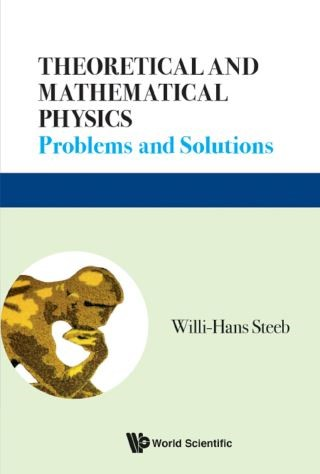## Theoretical and Mathematical Physics• Description
• Author
• Info
• Reviews

### Description

This updated and extended edition of the book combines the topics provided in the two parts of the previous editions as well as new topics. It is a comprehensive compilation covering most areas in mathematical and theoretical physics. The book provides a collection of problems together with their detailed solutions which will prove to be valuable to students as well as to researchers in the fields of mathematics, physics, engineering and other sciences.

Each chapter provides a short introduction with the relevant definitions and notations. All relevant definitions are given. The topics range in difficulty from elementary to advanced. Almost all problems are solved in detail and most of the problems are self-contained. Stimulating supplementary problems are also provided in each chapter. Students can learn important principles and strategies required for problem solving. Teachers will also find this text useful as a supplement, since important concepts and techniques are developed in the problems. Introductory problems for both undergraduate and advanced undergraduate students are provided. More advanced problems together with their detailed solutions are collected, to meet the needs of graduate students and researchers. Problems included cover new fields in theoretical and mathematical physics such as tensor product, Lax representation, Bäcklund transformation, soliton equations, Hilbert space theory, uncertainty relation, entanglement, spin systems, Lie groups, Bose system, Fermi systems differential forms, Lie algebra valued differential forms, metric tensor fields, Hirota technique, Painlevé test, Bethe ansatz, Yang–Baxter relation, wavelets, gauge theory, differential geometry, string theory, chaos, fractals, complexity, ergodic theory, etc. A number of software implementations are also provided.

Sample Chapter(s)
Chapter 1: Sums, Products and Discrete Fourier Transform

Contents:
• Sums, Products and Discrete Fourier Transform
• Transformations, Functions and Maps
• Algebraic and Transcendental Equations
• Vector and Matrix Calculus
• Matrices and Eigenvalue Problems
• Complex Analysis
• Integration
• Inequalities
• Optimization
• Ordinary Differential Equations
• Partial Differential Equations
• Functional Analysis, Hilbert Spaces and Wavelets
• Special Functions
• Generalized Functions
• Groups and Symmetries
• Combinatorics
• Number Theory
• Kronecker and Tensor Product
• Variational Calculus
• Lax Representation, Solitons and Bäcklund Transformations
• Hirota Technique and Painlevé Test
• Lie Groups and Lie Algebras
• Differential Forms and Matrix-Valued Differential Forms
• Metric Tensor Fields, Surfaces and Relativity
• Lie Derivative, Invariance and Killing Vector Fields
• Spin Systems
• Bose and Fermi Systems
• Gauge Transformation
• Bibliography
• Index